# End of Course Problem Set Math 804T Experimentation, Conjecture and Reasoning

Steven R. Dunbar

Date: Fall Semester 2007

1. Consider the sequence of Lucas numbers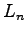, defined in Section 2.2, page 59, problem 10, and the sequence of Fibonacci numbers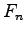. For 2 points, can you conjecture what the ratio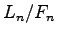seems to approach as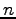gets larger? For 3 points can you provide some mathematical reasoning that justifies your conjecture with the methods of Section 2.2? (Hint: Use the result of Problem 12, page 59 and some of the ideas in of Problem 29, page 61.)

2. Make a new sequence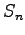which is the sum of the firstFibonacci numbers. For 2 points, make a table of values ofand make a conjecture about expressing this sequence in terms of the Fibonacci sequence. For 3 points, provide mathematical reasoning about why your conjecture is true.

3. Can you make a triangle with the following leg lengths: one leg is the sum of two consecutive odd integers, the other leg is the product of two consecutive odd integers, and the hypotenuse is two more than the product? Can you make a right triangle with those leg lengths? For two points, provide some experimentation and a conjecture, for 3 points, provide some mathematical reasoning which justifies your conjecture.

4. The cube can be inscribed in a sphere, that is, a sphere which touches the 8 vertices of the cube. What is the radius of that sphere which has an inscribed cube of side length 1? (For bonus points, not required, just for fun and extra points if you get it right! What is the radius of a sphere which has an inscribed tetrahedron of side length 1?)

5. A circle passes through the three vertices of an isosceles triangle that has two sides of length 3 and a base of length 2. What is the area of the circle? (Two points for a decimal approximation correct to two places, 3 points for mathematical reasoning that gives a mathematical answer.)

6. At a teaching council 4 math teachers, 3 English teachers and 3 foreign language teachers are to be seated in a row. How many seating arrangements are possible when teachers of the same subject are required to sit together?

7. A mother is holding a birthday party with several excited young children. She hasdistinctively wrapped party favors to give tochildren. She now has a headache, so she quickly hands out each favor package randomly without looking to see if the recipient already has a package. For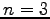,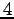, and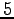, find the probability that each child gets a package.

8. A paper bag discovered at the back of a closet shelf is found to contain twelve old light bulbs. Five of them are 25-watt, six are burned out and three are both. Find the probability that a bulb drawn at random from the bag is burned out, given that it is 25-watt. Also find the probability that it is not 25-watt given that it is burned out.

9. Suppose that an insurance company classifies people into one of three classes - good risks, average risks and bad risks. The company records indicate that probabilities that good, average and bad risk persons will be involved in an accident over a 1-year span are respectively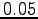,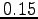and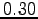. If 20% of the population are good risks, 50% are average risks and 30% are bad risks, what proportion of people have accidents in a given calendar year? If a policy holder Steve had no accidents in 2007, what is the probability that Steve is a good risk?

10. Write a short (one page or less) reflection on what was your favorite problem in the course, and why it was your favorite. Explain what you learned about mathematics and problem solving from your favorite problem.

End of Course Problem Set
Math 804T
Experimentation, Conjecture and Reasoning

This document was generated using the LaTeX2HTML translator Version 2002-2-1 (1.71)

Copyright © 1993, 1994, 1995, 1996, Nikos Drakos, Computer Based Learning Unit, University of Leeds.
Copyright © 1997, 1998, 1999, Ross Moore, Mathematics Department, Macquarie University, Sydney.

The command line arguments were:
latex2html end_of_course_fall07.tex

The translation was initiated by Steven Dunbar on 2007-12-14Steven R. Dunbar
Department of Mathematics and Statistics